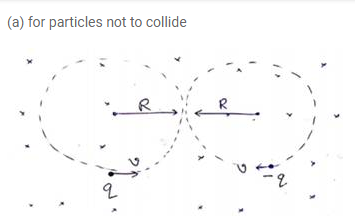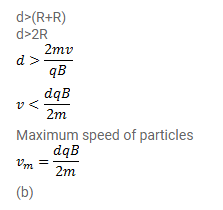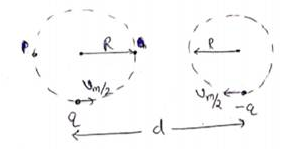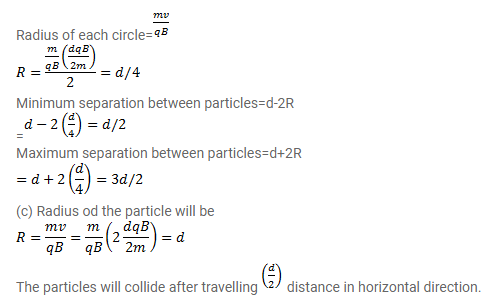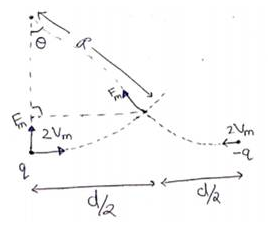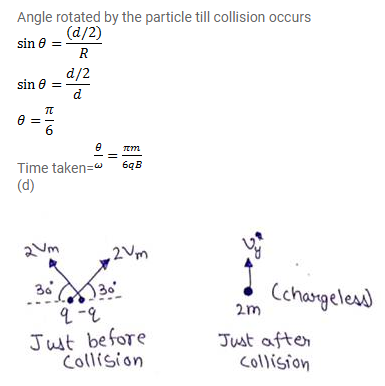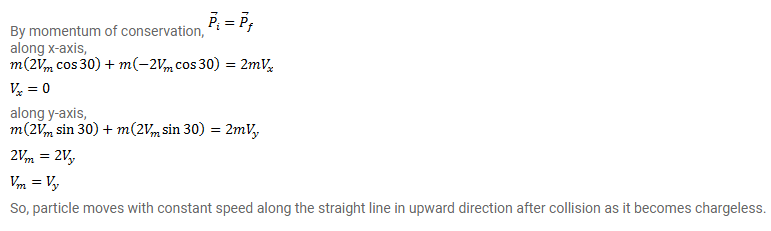# Two particles, each having mass m are placed at a separation d

Question:

Two particles, each having mass $m$ are placed at a separation $d$ in a uniform magnetic field $B$ as shown in figure. They have opposite charges of equal magnitude q. At time $t=0$, the particles are projected towards each other, each with a speed $v$. Suppose the Coulomb force between the charges is switched off.

(a) find the maximum value $v_{m}$ of the projection speed so that the two particles do not collide.

(b) What would be the minimum and maximum separation between the particles if $v=v_{m} / 2$ ?

(c) At what instant will a collision occur between the particles if $v=2 v_{m}$ ?

(d) Suppose $v=2 v_{m}$ and the collision between the particles is completely inelastic. Describe the motion after the collision.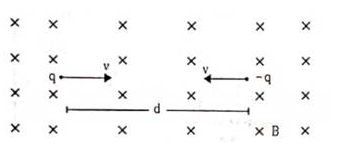Solution: